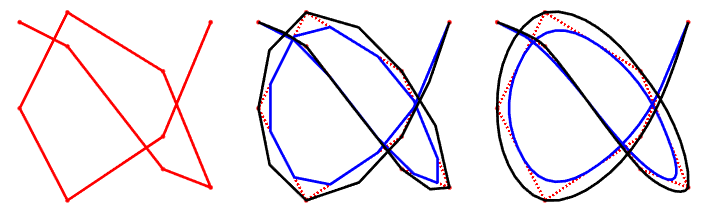Categories

# Tools for line drawings

Here’s a collection of useful tools for making line drawings with a pen plotter. I assume that the drawing is composed of lines, where each one is defined as a series of points connected with straight line segments. A point can be considered a degenerate line, with no segments.

## Bounding box intersection

If you have some shapes, the easiest way to check whether they could possibly intersect is by comparing their bounding boxes. First, you need to find the bounding boxes. That is, find the minimum and maximum positions along each axis. Then you can define the center of the bounding rectangle as $(x_0,y_0) = (x_{min}+x_{max})/2,(y_{min}+y_{max})/2$, and the width and height as $w= (x_{max}-x_{min}), \, h = (y_{max}-y_{min})$. Then the boxes overlap if two conditions are met: the absolute x-difference in their center positions is less than half the sum of their width, and the same idea for the y-difference. That is, if we have two bounding boxes $A$ and $B$, they overlap if the following statements are true:

$|A_{x,0}-B_{x,0}|\leq (w_A+w_B)/2 \\ |A_{y,0}-B_{y,0}|\leq (h_A+h_B)/2$

## Line segment intersection

Where do line segments intersect? Let’s call the segments $A$ and $B$. Label the start and end points with subscript 1 and 2, respectively. Parameterize the position along the segments with $t$, and for simplicity define $A_{x,2}-A_{x,1}=A_{\Delta x}$.

The first test can check the bounding boxes. Next, check if the lines can intersect via the cross product. If $(A_{\Delta x} B_{\Delta y} - A_{\Delta y} B_{\Delta x})=0$, then the lines are parallel, so no intersection.

Now, assuming that they are non-parallel, we have:

\begin{aligned} A_{x,1} + t_A A_{\Delta x} &= B_{x,1} + t_B B_{\Delta x} \\ A_{y,1} + t_A A_{\Delta y} &= B_{y,1} + t_B B_{\Delta y} \end{aligned}

Solve for $t_A$ and $t_B$:

\begin{aligned} t_A &= \frac{ B_{\Delta x}\left( A_{y,1} - B_{y,1} \right) + B_{\Delta y} \left( -A_{x,1}+ B_{x,1} \right) }{ A_{\Delta x} B_{\Delta y} -A_{\Delta y} B_{\Delta x} } \\ t_B &= \frac{ A_{\Delta x}\left( A_{y,1} -B_{y,1} \right) + A_{\Delta y} \left( -A_{x,1}+ B_{x,1} \right) }{ A_{\Delta x} B_{\Delta y} - A_{\Delta y} B_{\Delta x} } \end{aligned}

If $0 \leq t_A \leq 1$ and $0 \leq t_B \leq 1$, then the segments intersect. The intersection position can be found by substituting $t_A$ back into the original equations.

## Line Simplification

Do you have too many points on your curves? You don’t need to specify a point every millimeter when you have long, almost-straight lines. There are two common approaches for this sort of decimation with iterative point removal. The general idea is that you want to remove points that don’t contribute much. That is, see how much error would be created by removing points, and decide whether or not to remove some.

The first way, similar to the Ramer–Douglas–Peucker algorithm is to define the error as the distance from the new line segment to the removed point. The second, similar to Visvalingam’s algorithm, is to define the error as the area of the triangle formed by the new segment and the removed point.From left to right: Edges of the Mona Lisa, distance-based decimation, area-based decimation. The bottom row shows the original in blue, and decimated in red. Both decimated versions have 14% of the original number of points.

As the image above shows, both methods work fine, even with a lot of removed points. There are lots of other line simplification methods, but I like these.

## Removing hidden lines

Imagine that we have a whole pile of rectangular sheets of paper and we only want to draw the visible edges. This is the problem of hidden line removal (or hidden surface removal).

Asuming that each polygon (sheet of paper) is flat and parallel to the image plane, there are a couple basic ways to do this: compare intersections of line segments and discard the hidden ones, or use a bitmap to keep track of what is and isn’t visible. The first method gives exact answers, but can be quite costly. The second method gives an approximation, but can be much faster when there are many lines and shapes. I’ll talk about these more another time.

## Line Smoothing

Are your curves too jagged? Did you go too far in your simplification, and want to add some gentle curves? Here are some ideas.

A common, useful approach is Chaikin smoothing. This is an iterative procedure that, in the limit, produces the points of a quadratic spline. The basic idea is to take each line segment and squeeze is to half its length, then add segments between the newly separated regions.

Chaikin smoothing is great, but it acts like it doesn’t trust the vertices. If you’d rather the smooth curve passes through the vertices, try this modified version instead.Left is the unsmoothed path. The Chaikin smoothed path is in blue, and the vertex-trusting path is in black. The middle plot shows one iteration, and the right plot shows three.

Or if you mostly trust your lines, you can do Fourier-based smoothing. The general idea is to take uniformly spaced points along the length of your path, then use some frequency-based filtering via an FFT to remove the sharp kinks. For more details, read here.

## Line Sorting

If you’re drawing these lines on a computer, there is no need to sort the lines cleverly. However, if you plan to use a machine to draw them, you want to minimize the total time that the machine is moving. If you don’t optimize the line-drawing order, you could spent a lot of extra time watching the pen traveling in the air. The simplest method is the greedy one: each time you finish drawing a line, draw the closest undrawn line. There are lots of other approaches, and I talk about some of them in another post.

This site uses Akismet to reduce spam. Learn how your comment data is processed.